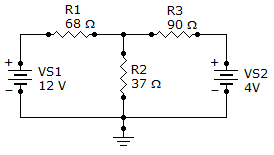# Electrical Engineering - Branch, Loop and Node Analyses - Discussion

Discussion Forum : Branch, Loop and Node Analyses - General Questions (Q.No. 14)
14.
What is the voltage drop across R3?112 mV
11.25 V
180 mV
1.80 V
Explanation:
No answer description is available. Let's discuss.
Discussion:
16 comments Page 1 of 2.

Geeta H said:   9 months ago
Thanks for explaining @Somesh.

Nireeksha said:   5 years ago
Here, we can use 4-va/90.

Clex said:   6 years ago
VA.4.18 is right. Further, how can be extracted 180mv?

Somesh said:   6 years ago
For first loop:
12= 68i' + 37(i' + i")
12= 105 i' + 37 i" -----------------------1

For 2nd loop:
4= 90 i" + 37(i' + i")
4= 37 i' + 127 i" ----------------------------2

Solve equations 1 and 2 we get;

i' =114mA,
i" = 2mA,

Vr3 = i" X R,
Vr3 = 2mA X 90,
Vr3 = 180mV.

Bikram said:   7 years ago
I have a question. When we have to use +ve or -ve sign in KCL nodal analysis equation?

Nehal patel said:   7 years ago
r1 + r2 + r3 - (vs1 + vs2) = r3.

Paul said:   7 years ago
I'm still not the wiser. Anyone help me?

ALI said:   8 years ago
We consider the print between R1, R2 and R3 = VA.

VA-12/86+VA-0/37+VA-4/90 = 0.

(90*37)*VA-(90*37)*12+(86*90)*VA+(37*86)*VA-(37*86)*4/(37*90*86) = 0.

11966 VA-50024 = 0.

11966 VA = 50024.

VA = 4.18.

Pratik soni said:   8 years ago